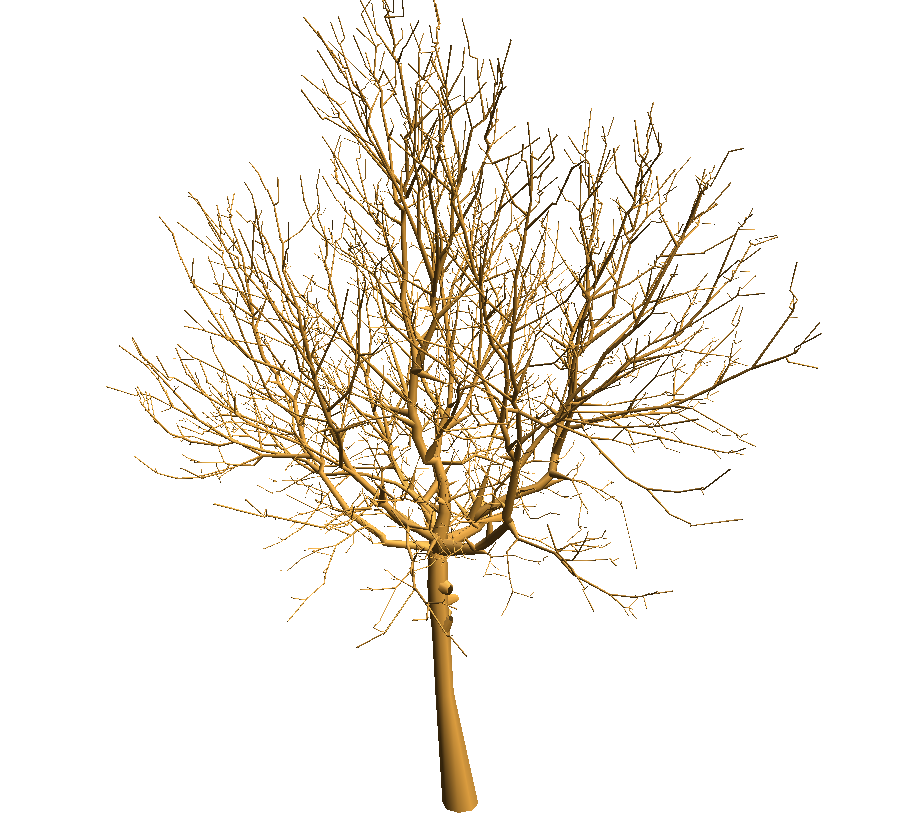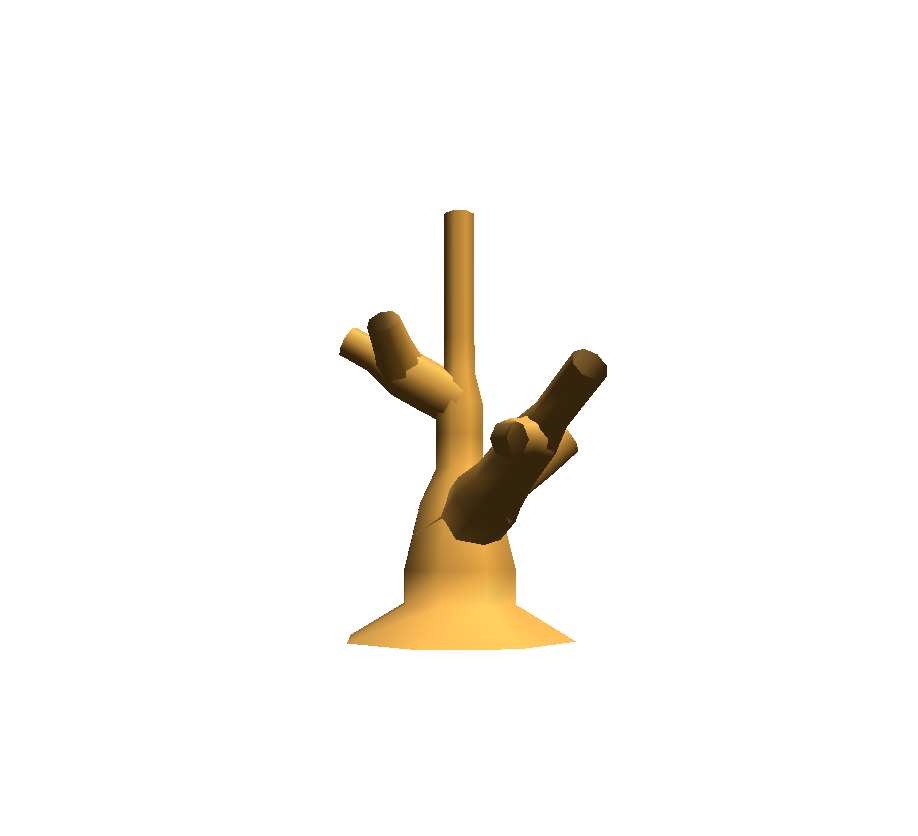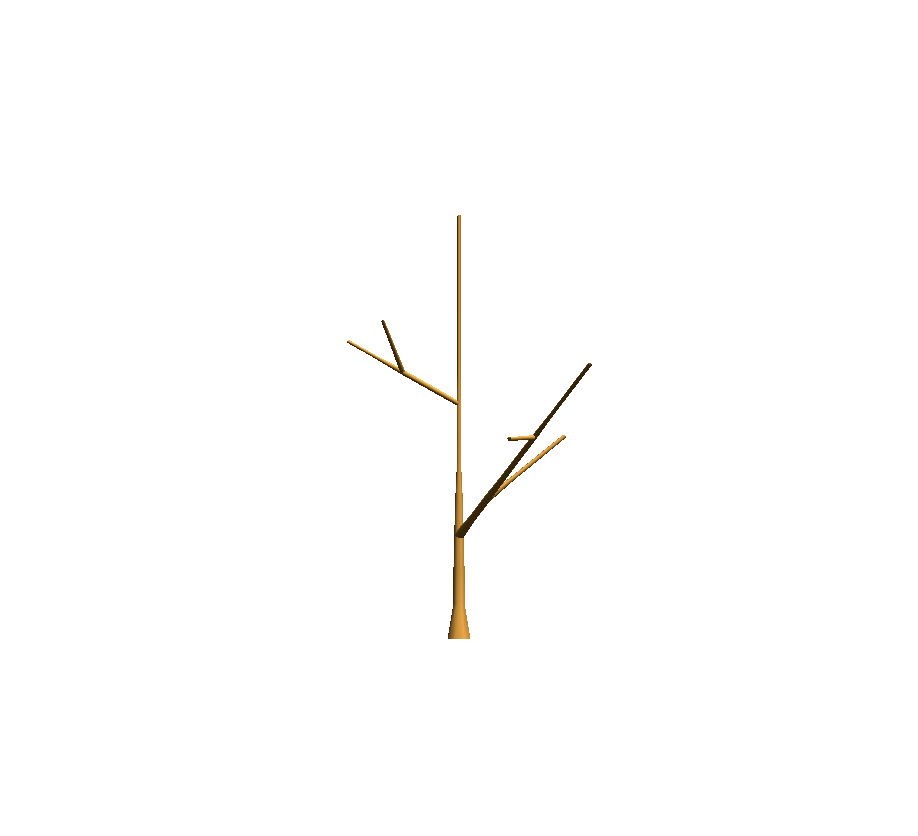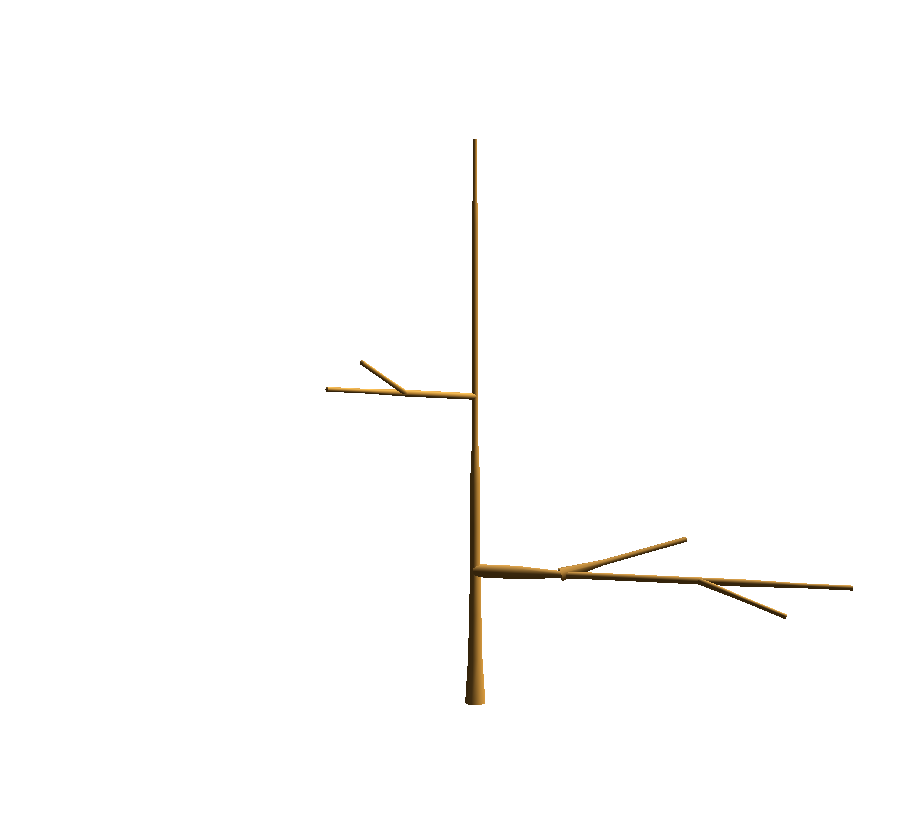# 6. PlantFrame (3D reconstruction of plant architecture)¶

Section contents

In this section, we introduce the PlantFrame vocabulary that we use through-out vplants and give a series of examples.

## 6.1. The problem setting¶

PlantFrame is a method to compute the geometry of each organ of a Plant Architecture. Geoemtrical data is associated to some vertices of the architecture (aka MTG). But often, geometrical information is missing on some vertex. Constraints have to be solved to compute missing values.

The stages of the PlantFrame are:
1. Insert a scale at the axis level.
2. Project all the constraints at the finer scale.
3. Apply different Knowledge Sources (i.e. KS) on the MTG to compute the values at some nodes.
4. Solve the constraints.
5. Visualise the geometry using a 3D Turtle.

## 6.2. Where are the data?¶

The tutorial package comes with a few datasets. The data are in share/data/PlantFrame directory from the root.

>>> import openalea.mtg
>>> from openalea.deploy.shared_data import shared_data
>>> import vplants.tutorial
>>> data = shared_data(vplants.tutorial)/'PlantFrame'


## 6.3. Visualisation of a digitized Tree¶

First, we load the digitized Walnut noylum2.mtg

>>> from openalea.mtg import *
>>> g = MTG(data/'noylum2.mtg')


Then, a file containing a set of default geometric parameters is loaded to build a DressingData (walnut.drf )

>>> drf = data/'walnut.drf'
>>> dressing_data = dresser.dressing_data_from_file(drf)


Another solution is to create the default parameters directly

::
>>> dressing_data = plantframe.DressingData(DiameterUnit=10)


Geometric parameters are missing. How to compute them? Use the PlantFrame, a geometric solver working on multiscale tree structure.

Create the solver and solve the problem

>>> pf = plantframe.PlantFrame(g,
TopDiameter='TopDia',
DressingData = dressing_data)


Visualise the plant in 3D

>>> pf.plot(gc=True)## 6.4. Simple visualisation of a monopodial plant¶

First, we load the MTG monopodial_plant.mtg

>>> from openalea.mtg import *
>>> g = MTG(data/'monopodial_plant.mtg')

>>> def coloring(mtg, vertex):
try:
mtg.property('diam')[vertex]
return "g"
except: return "r"

>>> def legend(mtg, vertex):
try:
return "diam: "+str(mtg.property('diam')[vertex])
except: return "diam: NA"

>>> def label(mtg, vertex):
return mtg.label(vertex)

>>> g.plot(roots=4, node=dict(fc=coloring, label=label, legend=legend), prog="dot")>>> def legend(mtg, vertex):
try:
return "diam: "+str(mtg.property('diam')[vertex])
except: return "diam: NA"

>>> g.plot(roots=4, node=dict(fc=coloring, label=label, legend=legend), prog="dot")The mtg monopodial_plant.mtg is loaded. To draw it, just run:

>>> pf = plantframe.PlantFrame(g, TopDiameter='diam')
>>> pf.plot()You can also define a function to compute the diameter:

>>> def diam(v):
d = g.node(v).diam
return d/10. if d else None
>>> pf = plantframe.PlantFrame(g, TopDiameter=diam)
>>> pf.plot()The diameter is defined for each vertex of the MTG. To take into account the diameter, we have to define a visitor function.

diam = g.property('diam')

def visitor(g, v, turtle):
if g.edge_type(v) == '+':
angle = 90 if g.order(v) == 1 else 30
turtle.down(angle)
turtle.setId(v)
if v in diam:
turtle.setWidth(diam[v]/2.)
turtle.F(10)
turtle.rollL()

pf = plantframe.PlantFrame(g)
pf.plot(g, visitor=visitor)